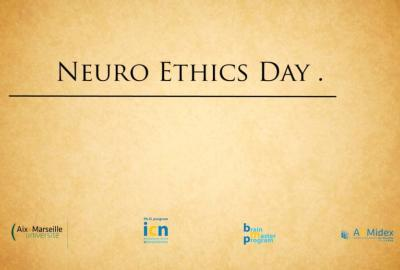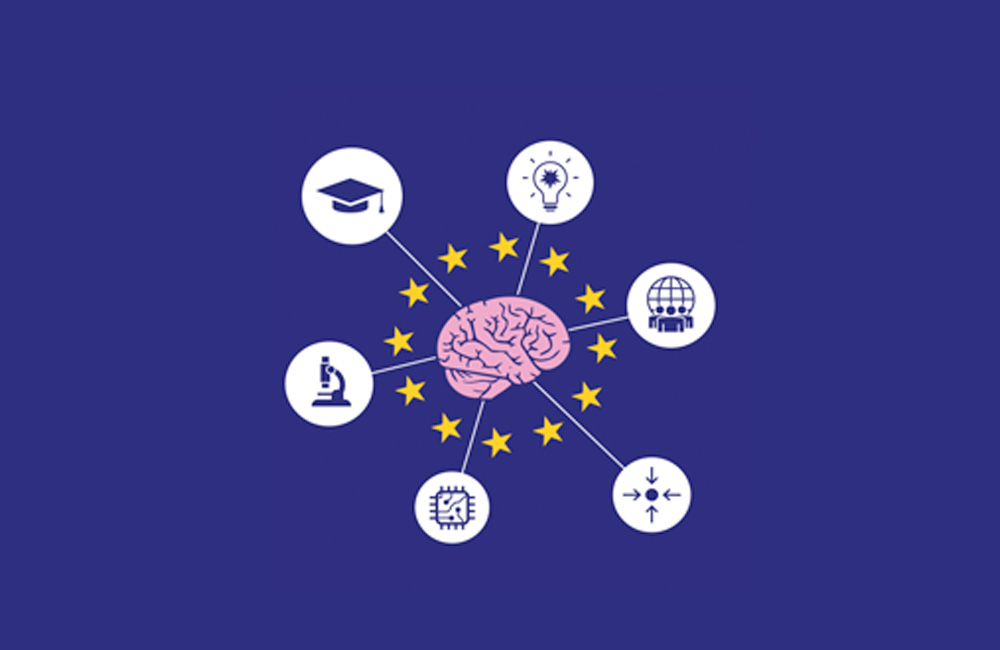# Module 5: Calcium Imaging

Category
Level
Intermediate

In this course, you will learn about working with calcium-imaging data, including image processing to remove background "blur", identifying cells based on threshold spatial contiguity, time-series filtering, and principal component analysis (PCA). The MATLAB code shows data animations, capabilities of the image processing toolbox, and PCA.

Course Features
Videos
Tutorials
Exercises
Code / Datasets
Lessons of this Course
1
1
Duration:
5:02

This lesson introduces various methods in MATLAB useful for dealing with data generated by calcium imaging.

2
2
Duration:
15:01

This tutorial demonstrates how to use MATLAB to generate and visualize animations of calcium fluctuations over time.

3
3
Duration:
5:15

This tutorial instructs users how to use MATLAB to programmatically convert data from cells to a matrix.

4
4
Duration:
17:08

In this tutorial, users will learn how to identify and remove background noise, or "blur", an important step in isolating cell bodies from image data.

5
5
Duration:
11:23

This lesson teaches users how MATLAB can be used to apply image processing techniques to identify cell bodies based on contiguity.

6
6
Duration:
22:41

This tutorial demonstrates how to extract the time course of calcium activity from each clusters of neuron somata, and store the data in a MATLAB matrix.

7
7
Duration:
17:19

This lesson demonstrates how to use MATLAB to implement a multivariate dimension reduction method, PCA, on time series data.

##### Recent courses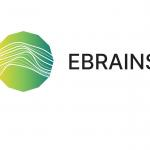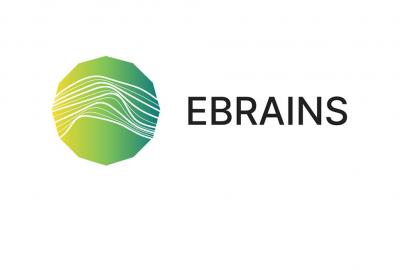#### Open Data in Neuroscience: Data Sharing in EBRAINS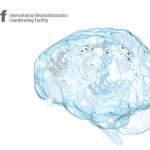#### Introduction to Computational Neuroscience